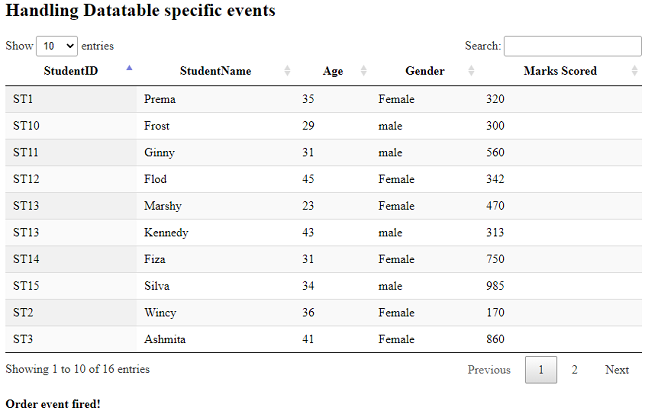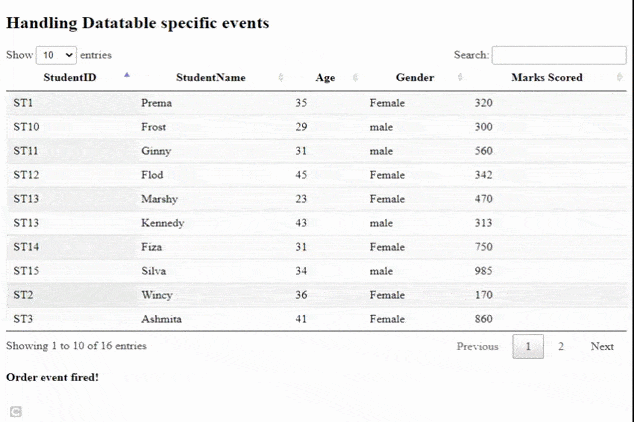# How to handle DataTable specific events using jQuery DataTable plugin ?

• Last Updated : 14 Jan, 2021

DataTables are a modern jQuery plugin for adding interactive and advanced controls to HTML tables for our webpage. DataTables is a simple-to-use plug-in with a variety of options for the developer’s custom changes. The common features of the DataTable plugin are pagination, searching, sorting, and multiple column ordering.

In this article, we will learn to handle DataTable specific order and paging events. Datatables also gives back many custom events.

Hey geek! The constant emerging technologies in the world of web development always keeps the excitement for this subject through the roof. But before you tackle the big projects, we suggest you start by learning the basics. Kickstart your web development journey by learning JS concepts with our JavaScript Course. Now at it's lowest price ever!

The pre-compiled files which are needed to implement the codes are

CSS:

`https://cdn.datatables.net/1.10.22/css/jquery.dataTables.min.css`

JavaScript :

`//cdn.datatables.net/1.10.22/js/jquery.dataTables.min.js`

Approach: To handle DataTable specific events dt namespace is used.  All the events are triggered with the dt namespace. The datatable on() method is used which automatically appends dt namespace. Order event is automatically fired when the data in the table is ordered. Paging event is fired whenever pagination feature is used.

HTML table is designed with student’s data with id, name , age, gender and marks as their fields. HTML div with id’s orderResultID and pagingResultID is created to show a message whenever the order and paging events are fired.

Example: The following example code demonstrates how to handle functions whenever the DataTable specific events are fired. A simple message is shown below the table. The developer can write functions according to the application’s need.

## HTML

 ```<``html``>`` ` `<``head``>``    ``<``meta` `content="``initial-scale``=``1``, ``        ``maximum-scale``=``1``, ``user-scalable``=``0``"``        ``name``=``"viewport"` `/>`` ` `    ``<``meta` `name``=``"viewport"` `content``=``"width=device-width"` `/>`` ` `    ````    ``<``link` `rel``=``"stylesheet"` `href``=``"https://cdn.datatables.net/1.10.22/css/jquery.dataTables.min.css"` `/>`` ` `    ````    ``<``script` `type``=``"text/javascript"` `        ``src``=``"https://code.jquery.com/jquery-3.5.1.js"``>``    ```` ` `    ````    ``<``script` `type``=``"text/javascript"` `src``=``"https://cdn.datatables.net/1.10.22/js/jquery.dataTables.min.js"``>``    `````` ` `<``body``>``    ``<``h2``>Handling Datatable specific events`` ` `    ````    ``<``table` `id``=``"tableID"` `class``=``"display"` `        ``style``=``"width:100%"``>``        ``<``thead``>``            ``<``tr``>``                ``<``th``>StudentID``                ``<``th``>StudentName``                ``<``th``>Age``                ``<``th``>Gender``                ``<``th``>Marks Scored``            ````        ```` ` `        ``<``tbody``>``            ``<``tr``>``                ``<``td``>ST1``                ``<``td``>Prema``                ``<``td``>35``                ``<``td``>Female``                ``<``td``>320``            ````            ``<``tr``>``                ``<``td``>ST2``                ``<``td``>Wincy``                ``<``td``>36``                ``<``td``>Female``                ``<``td``>170``            ````            ``<``tr``>``                ``<``td``>ST3``                ``<``td``>Ashmita`` ` `                ``<``td``>41``                ``<``td``>Female``                ``<``td``>860``            ````            ``<``tr``>``                ``<``td``>ST4``                ``<``td``>Kelina``                ``<``td``>32``                ``<``td``>Female``                ``<``td``>433``            ````            ``<``tr``>``                ``<``td``>ST5``                ``<``td``>Satvik``                ``<``td``>41``                ``<``td``>male``                ``<``td``>162``            ````            ``<``tr``>``                ``<``td``>ST6``                ``<``td``>William``                ``<``td``>37``                ``<``td``>Female``                ``<``td``>372``            ````            ``<``tr``>``                ``<``td``>ST7``                ``<``td``>Chandan``                ``<``td``>31``                ``<``td``>male``                ``<``td``>375``            ````            ``<``tr``>``                ``<``td``>ST8``                ``<``td``>David``                ``<``td``>45``                ``<``td``>male``                ``<``td``>327``            ````            ``<``tr``>``                ``<``td``>ST9``                ``<``td``>Harry``                ``<``td``>29``                ``<``td``>male``                ``<``td``>205``            ````            ``<``tr``>``                ``<``td``>ST10``                ``<``td``>Frost``                ``<``td``>29``                ``<``td``>male``                ``<``td``>300``            ````            ``<``tr``>``                ``<``td``>ST11``                ``<``td``>Ginny``                ``<``td``>31``                ``<``td``>male``                ``<``td``>560``            ````            ``<``tr``>``                ``<``td``>ST12``                ``<``td``>Flod``                ``<``td``>45``                ``<``td``>Female``                ``<``td``>342``            ````            ``<``tr``>``                ``<``td``>ST13``                ``<``td``>Marshy``                ``<``td``>23``                ``<``td``>Female``                ``<``td``>470``            ````            ``<``tr``>``                ``<``td``>ST13``                ``<``td``>Kennedy``                ``<``td``>43``                ``<``td``>male``                ``<``td``>313``            ````            ``<``tr``>``                ``<``td``>ST14``                ``<``td``>Fiza``                ``<``td``>31``                ``<``td``>Female``                ``<``td``>750``            ````            ``<``tr``>``                ``<``td``>ST15``                ``<``td``>Silva``                ``<``td``>34``                ``<``td``>male``                ``<``td``>985``            ````        ````    ````    ``<``br` `/>`` ` `    ``<``div` `id``=``"orderResultID"``>``    ``<``div` `id``=``"pagingResultID"``>`` ` `    ``<``script``>`` ` `            ``/* Initialization of datatables */``            ``\$(document).ready(function () {`` ` `                ``/* events like order and paging are fired */``                    ``\$('#tableID')``                        ``.on('order.dt', function () {``                            ``\$("#orderResultID").show()``                            ``.html("<``b``>Order event fired! ");``                        ``}``                        ``).DataTable();`` ` `                ``\$('#tableID').on('page.dt', function () {``                    ``\$("#pagingResultID").show()``                    ``.html("<``b``>Paging event fired! ");``                ``}``                ``).DataTable();``            ``});``    `````` ` ``

Output:

Order event:Paging event:My Personal Notes arrow_drop_up